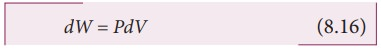Home | | Physics 11th std | Work done in volume changes - Internal Energy (U)

# Work done in volume changes - Internal Energy (U)

Consider a gas contained in the cylinder fitted with a movable piston.

Work done in volume changes

Consider a gas contained in the cylinder fitted with a movable piston. Suppose the gas is expanded quasi-statically by pushing the piston by a small distance dx as shown in Figure 8.21. Since the expansion occurs quasi-statically the pressure, temperature and internal energy will have unique values at every instant.

The small work done by the gas on the pistonThe force exerted by the gas on the piston F = PA. Here A is area of the piston and P is pressure exerted by the gas on the piston.Equation (8.14) can be rewritten asBut Adx = dV= change in volume during this expansion process.

So the small work done by the gas during the expansion is given byNote here that is positive since the volume is increased. Here, is positive.

In general the work done by the gas by increasing the volume from Vi to Vf is given bySuppose if the work is done on the system, then Vi > Vf . Then, W is negative.

Note here the pressure P is inside the integral in equation (8.17). It implies that while the system is doing work, the pressure need not be constant. To evaluate the integration we need to first express the pressure as a function of volume and temperature using the equation of state.

Study Material, Lecturing Notes, Assignment, Reference, Wiki description explanation, brief detail
11th Physics : UNIT 8 : Heat and Thermodynamics : Work done in volume changes - Internal Energy (U) |